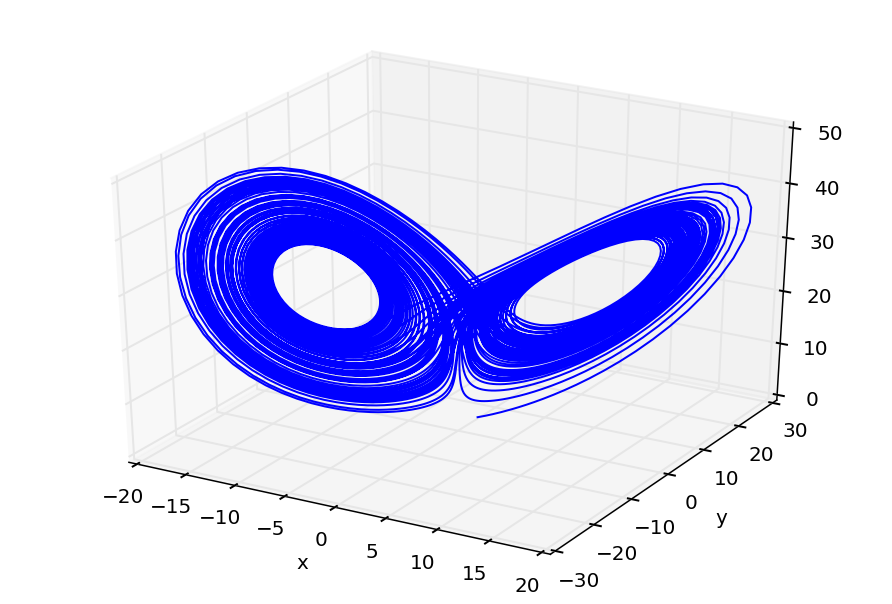# Initial imports¶

In :
%pylab inline

Welcome to pylab, a matplotlib-based Python environment [backend: module://IPython.kernel.zmq.pylab.backend_inline].

In :
from matplotlib import pyplot as plt
import numpy as np

from scipy.integrate import odeint

from colorline import colorline

In :
# Display the help for the odeint function:
# The output can be shortened or suppressed by clicking or double clicking on the region to the left of the output

help(odeint)

Help on function odeint in module scipy.integrate.odepack:

odeint(func, y0, t, args=(), Dfun=None, col_deriv=0, full_output=0, ml=None, mu=None, rtol=None, atol=None, tcrit=None, h0=0.0, hmax=0.0, hmin=0.0, ixpr=0, mxstep=0, mxhnil=0, mxordn=12, mxords=5, printmessg=0)
Integrate a system of ordinary differential equations.

Solve a system of ordinary differential equations using lsoda from the
FORTRAN library odepack.

Solves the initial value problem for stiff or non-stiff systems
of first order ode-s::

dy/dt = func(y,t0,...)

where y can be a vector.

Parameters
----------
func : callable(y, t0, ...)
Computes the derivative of y at t0.
y0 : array
Initial condition on y (can be a vector).
t : array
A sequence of time points for which to solve for y.  The initial
value point should be the first element of this sequence.
args : tuple, optional
Extra arguments to pass to function.
Dfun : callable(y, t0, ...)
Gradient (Jacobian) of func.
col_deriv : bool, optional
True if Dfun defines derivatives down columns (faster),
otherwise Dfun should define derivatives across rows.
full_output : bool, optional
True if to return a dictionary of optional outputs as the second output
printmessg : bool, optional
Whether to print the convergence message

Returns
-------
y : array, shape (len(t), len(y0))
Array containing the value of y for each desired time in t,
with the initial value y0 in the first row.
infodict : dict, only returned if full_output == True

=======  ============================================================
key      meaning
=======  ============================================================
'hu'     vector of step sizes successfully used for each time step.
'tcur'   vector with the value of t reached for each time step.
(will always be at least as large as the input times).
'tolsf'  vector of tolerance scale factors, greater than 1.0,
computed when a request for too much accuracy was detected.
'tsw'    value of t at the time of the last method switch
(given for each time step)
'nst'    cumulative number of time steps
'nfe'    cumulative number of function evaluations for each time step
'nje'    cumulative number of jacobian evaluations for each time step
'nqu'    a vector of method orders for each successful step.
'imxer'  index of the component of largest magnitude in the
weighted local error vector (e / ewt) on an error return, -1
otherwise.
'lenrw'  the length of the double work array required.
'leniw'  the length of integer work array required.
'mused'  a vector of method indicators for each successful time step:
1: adams (nonstiff), 2: bdf (stiff)
=======  ============================================================

Other Parameters
----------------
ml, mu : int, optional
If either of these are not None or non-negative, then the
Jacobian is assumed to be banded.  These give the number of
lower and upper non-zero diagonals in this banded matrix.
For the banded case, Dfun should return a matrix whose
columns contain the non-zero bands (starting with the
lowest diagonal).  Thus, the return matrix from Dfun should
have shape len(y0) * (ml + mu + 1) when ml >=0 or mu >=0.
rtol, atol : float, optional
The input parameters rtol and atol determine the error
control performed by the solver.  The solver will control the
vector, e, of estimated local errors in y, according to an
inequality of the form max-norm of (e / ewt) <= 1,
where ewt is a vector of positive error weights computed as
ewt = rtol * abs(y) + atol.
rtol and atol can be either vectors the same length as y or scalars.
Defaults to 1.49012e-8.
tcrit : ndarray, optional
Vector of critical points (e.g. singularities) where integration
care should be taken.
h0 : float, (0: solver-determined), optional
The step size to be attempted on the first step.
hmax : float, (0: solver-determined), optional
The maximum absolute step size allowed.
hmin : float, (0: solver-determined), optional
The minimum absolute step size allowed.
ixpr : bool, optional
Whether to generate extra printing at method switches.
mxstep : int, (0: solver-determined), optional
Maximum number of (internally defined) steps allowed for each
integration point in t.
mxhnil : int, (0: solver-determined), optional
Maximum number of messages printed.
mxordn : int, (0: solver-determined), optional
Maximum order to be allowed for the non-stiff (Adams) method.
mxords : int, (0: solver-determined), optional
Maximum order to be allowed for the stiff (BDF) method.

--------
ode : a more object-oriented integrator based on VODE.
quad : for finding the area under a curve.



# Integrating ODEs using odeint¶

The function odeint, which is found in the integrate submodule of the scipy package, integrates ordinary differential equations of the form $$\newcommand{\yy}{\mathbf{y}} \newcommand{\ff}{\mathbf{f}} \frac{\mathrm{d} \yy}{\mathrm{d}t} = \ff(\yy, t),$$where $\yy$ is a vector in $\mathbb{R}^n$, or $$\dot{\yy} = \ff(\yy, t).$$ That is, it provides a numerical solution of the initial value problem given by this differential equation, together with an initial condition $$\yy(t = t_0) = \yy_0,$$ returning the solution curve (or trajectory) $\yy(t)$ as a function of time $t$, within a certain (finite!) range of values of $t$.

Writing $\yy = (y_1, \ldots, y_n)$, we have a system of the form \begin{align} \dot{y_1} &= f_1(y_1, \ldots, y_n, t) \\ \dot{y_2} &= f_2(y_1, \ldots, y_n, t) \\ &\ldots \\ \dot{y_n} &= f_n(y_1, \ldots, y_n, t) \end{align}

Note that in fact, any ordinary (system of) ordinary differential equation(s) can be written in this form by defining new variables to represent higher-order derivatives,
and incorporating them into the vector $\yy$ of variables.

To use odeint, we must define at least the Python function f which implements the mathematical function $\ff$. It must take a vector y and a time t, and return the new vector f(y,t).

## 1D equations¶

The simplest case is when the spatial dimension is $n=1$. For example, we could wish to solve the ODE $\dot{y} = ay,$ whose exact solution is, of course, $y(t) = y_0 \exp(a t)$.

In :
a = 2

def f(y, t):
return a*y


We must provide an initial condition for $y$, for example at $t=0$:

In :
y0 = 1.0


and an array of values of $t$ where we wish to have output:

In :
t_output = np.arange(0, 6, 0.1)
print t_output

[ 0.   0.1  0.2  0.3  0.4  0.5  0.6  0.7  0.8  0.9  1.   1.1  1.2  1.3  1.4
1.5  1.6  1.7  1.8  1.9  2.   2.1  2.2  2.3  2.4  2.5  2.6  2.7  2.8  2.9
3.   3.1  3.2  3.3  3.4  3.5  3.6  3.7  3.8  3.9  4.   4.1  4.2  4.3  4.4
4.5  4.6  4.7  4.8  4.9  5.   5.1  5.2  5.3  5.4  5.5  5.6  5.7  5.8  5.9]


We pass the information to the odeint function to perform the integration:

In :
y_result = odeint(f, y0, t_output)
y_result = y_result[:, 0]  # convert the returned 2D array to a 1D array


Now we can plot the results, together with the exact solution:

In :
#plt.plot(t_output, y_result)
colorline(t_output, y_result)
plt.plot(t_output, y0 * np.exp(a * t_output))

Out:
[<matplotlib.lines.Line2D at 0x114547310>]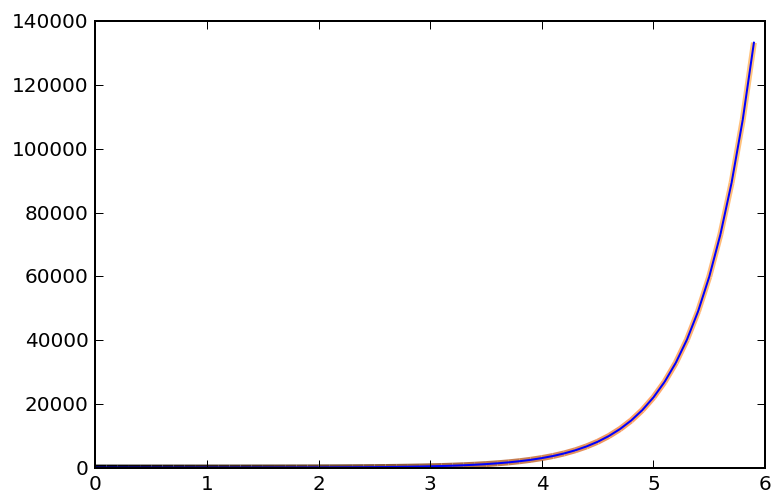We can calculate the absolute error between the numerically-calculated result and the exact solution:

In :
plt.plot(t_output, np.abs(y_result - y0 * np.exp(a * t_output)))

Out:
[<matplotlib.lines.Line2D at 0x1144ef410>]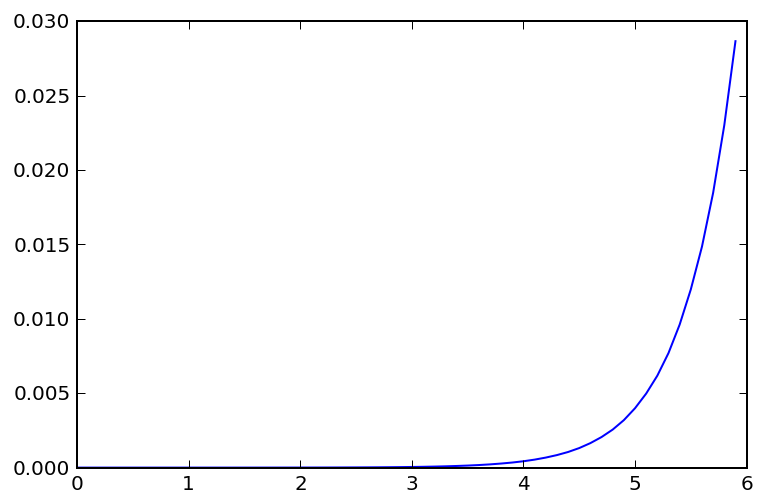## 2D equations¶

Now let's consider a simple 2nd-order ODE, that of the harmonic oscillator: $$\ddot{x} + \omega^2 x = 0.$$ To integrate this equation, we must rewrite it as a system of first-order ODEs by introducing a new variable $y := \dot{x}$, so that the system becomes \begin{align} \dot{x} &= y \\ \dot{y} &= - \omega^2 x \end{align}

Writing the vector $\yy = (x,y)$, we obtain the standard form $\dot{\yy} = \ff(\yy),$ where $\ff$ is given by $$\ff \left[ \begin{pmatrix} x \\ y \end{pmatrix} \right] = \begin{pmatrix} f_1 \left[ \begin{pmatrix} x \\ y \end{pmatrix} \right] \\ f_2 \left[ \begin{pmatrix} x \\ y \end{pmatrix} \right] \end{pmatrix} = \begin{pmatrix} y \\ -\omega^2 x \end{pmatrix}$$ That is, the functions $f_i$ are just the right-hand sides of each of the equations in the original system. We now implement this function $\ff$ directly as a function f in Python. Remember that it must also take t as a second variable, even if $\ff$ is not explicitly a function of $t$:

In :
omega_squared = 4

def harmonic(x_vec, t):
x, y = x_vec  # tuple unpacking

return [y, -omega_squared*x]

y0 = [0.7, 0.5]

t_output = np.arange(0, 5, 0.1)

y_result = odeint(harmonic, y0, t_output)

In :
#plt.figure(figsize = (5,5))

fig, (ax1, ax2, ax3) = plt.subplots(ncols = 3, figsize=(10,10))

xx, yy = y_result.T  # extract x and y cols

#ax1.plot(xx, yy)
plt.sca(ax1)
colorline(xx, yy, cmap='jet')
ax1.axis('scaled')

#ax2.plot(t_output, xx)
plt.sca(ax2)
colorline(t_output, xx, cmap='cool')
ax2.axis('scaled')

ax3.plot(t_output, yy)
ax3.axis('scaled')

plt.tight_layout()
plt.show()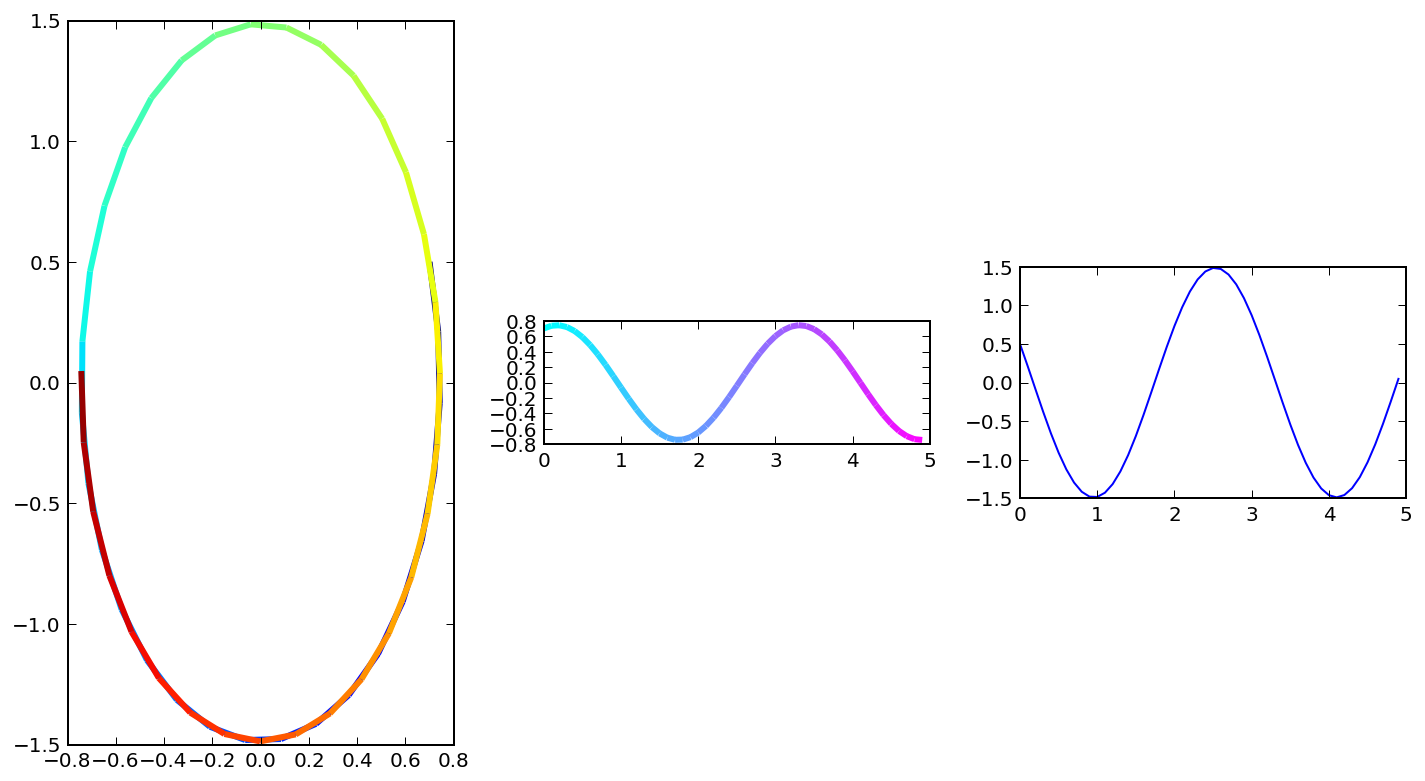In :
x = np.linspace(-1.0, 1.0, 10)
XX, YY = np.meshgrid(x, x)

In :
k = omega_squared
plt.quiver(XX, YY, YY, -k*XX, pivot='middle')
plt.axis('equal')

Out:
(-1.0, 1.0, -1.0, 1.0)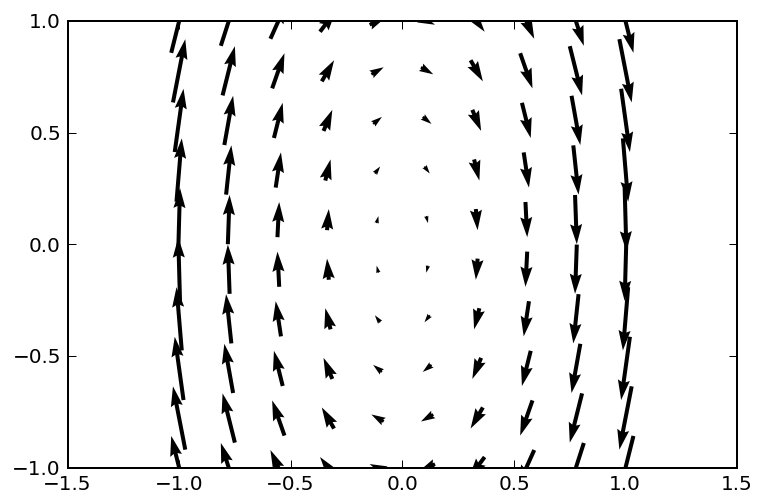In :
plt.streamplot(XX, YY, YY, -k*XX)
plt.quiver(XX, YY, YY, -k*XX, pivot = 'middle')
plt.axis('equal')

Out:
(-1.5, 1.0, -1.0, 1.0)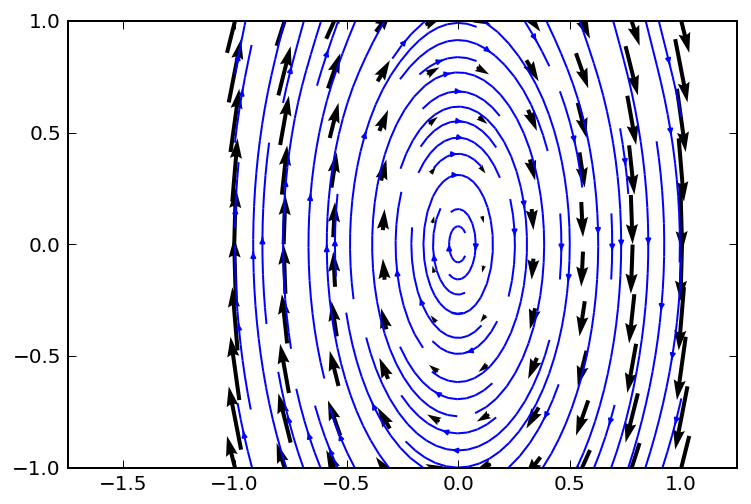Now let's try with a matrix:

In :
MM = np.array([[-1.5, 1.], [-1., -1.0]])

def matrix(x_vec, t):

return np.dot(MM, x_vec)

y0 = [1, 1]

t_output = np.arange(0, 10, 0.1)
y_result = odeint(matrix, y0, t_output)

In :
fig, (ax1, ax2, ax3) = plt.subplots(ncols = 3)

xx, yy = y_result.T  # extract x and y cols

ax1.plot(xx, yy)
ax1.axis('scaled')

ax2.plot(t_output, xx)
ax2.axis('scaled')

ax3.plot(t_output, yy)
ax3.axis('scaled')

plt.tight_layout()
plt.show()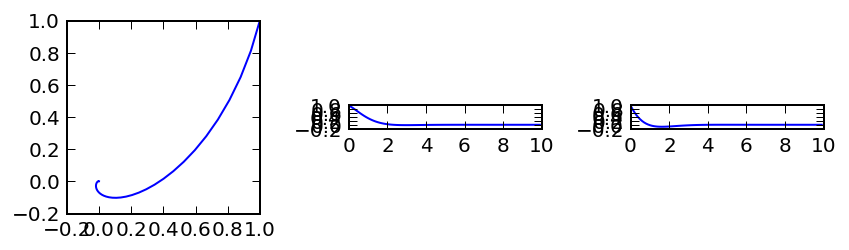# Using the ode interface¶

An alternative interface is ode.

In :
from scipy.integrate import ode

In :
help(ode)

Help on class ode in module scipy.integrate._ode:

class ode(__builtin__.object)
|  A generic interface class to numeric integrators.
|
|  Solve an equation system :math:y'(t) = f(t,y) with (optional) jac = df/dy.
|
|  Parameters
|  ----------
|  f : callable f(t, y, *f_args)
|      Rhs of the equation. t is a scalar, y.shape == (n,).
|      f_args is set by calling set_f_params(*args).
|      f should return a scalar, array or list (not a tuple).
|  jac : callable jac(t, y, *jac_args)
|      Jacobian of the rhs, jac[i,j] = d f[i] / d y[j].
|      jac_args is set by calling set_f_params(*args).
|
|  Attributes
|  ----------
|  t : float
|      Current time.
|  y : ndarray
|      Current variable values.
|
|  --------
|  odeint : an integrator with a simpler interface based on lsoda from ODEPACK
|  quad : for finding the area under a curve
|
|  Notes
|  -----
|  Available integrators are listed below. They can be selected using
|  the set_integrator method.
|
|  "vode"
|
|      Real-valued Variable-coefficient Ordinary Differential Equation
|      solver, with fixed-leading-coefficient implementation. It provides
|      implicit Adams method (for non-stiff problems) and a method based on
|      backward differentiation formulas (BDF) (for stiff problems).
|
|      Source: http://www.netlib.org/ode/vode.f
|
|      .. warning::
|
|         This integrator is not re-entrant. You cannot have two ode
|         instances using the "vode" integrator at the same time.
|
|      This integrator accepts the following parameters in set_integrator
|      method of the ode class:
|
|      - atol : float or sequence
|        absolute tolerance for solution
|      - rtol : float or sequence
|        relative tolerance for solution
|      - lband : None or int
|      - rband : None or int
|        Jacobian band width, jac[i,j] != 0 for i-lband <= j <= i+rband.
|        Setting these requires your jac routine to return the jacobian
|        in packed format, jac_packed[i-j+lband, j] = jac[i,j].
|      - method: 'adams' or 'bdf'
|        Which solver to use, Adams (non-stiff) or BDF (stiff)
|      - with_jacobian : bool
|        Whether to use the jacobian
|      - nsteps : int
|        Maximum number of (internally defined) steps allowed during one
|        call to the solver.
|      - first_step : float
|      - min_step : float
|      - max_step : float
|        Limits for the step sizes used by the integrator.
|      - order : int
|        Maximum order used by the integrator,
|        order <= 12 for Adams, <= 5 for BDF.
|
|  "zvode"
|
|      Complex-valued Variable-coefficient Ordinary Differential Equation
|      solver, with fixed-leading-coefficient implementation.  It provides
|      implicit Adams method (for non-stiff problems) and a method based on
|      backward differentiation formulas (BDF) (for stiff problems).
|
|      Source: http://www.netlib.org/ode/zvode.f
|
|      .. warning::
|
|         This integrator is not re-entrant. You cannot have two ode
|         instances using the "zvode" integrator at the same time.
|
|      This integrator accepts the same parameters in set_integrator
|      as the "vode" solver.
|
|      .. note::
|
|          When using ZVODE for a stiff system, it should only be used for
|          the case in which the function f is analytic, that is, when each f(i)
|          is an analytic function of each y(j).  Analyticity means that the
|          partial derivative df(i)/dy(j) is a unique complex number, and this
|          fact is critical in the way ZVODE solves the dense or banded linear
|          systems that arise in the stiff case.  For a complex stiff ODE system
|          in which f is not analytic, ZVODE is likely to have convergence
|          failures, and for this problem one should instead use DVODE on the
|          equivalent real system (in the real and imaginary parts of y).
|
|  "lsoda"
|
|      Real-valued Variable-coefficient Ordinary Differential Equation
|      solver, with fixed-leading-coefficient implementation. It provides
|      automatic method switching between implicit Adams method (for non-stiff
|      problems) and a method based on backward differentiation formulas (BDF)
|      (for stiff problems).
|
|      Source: http://www.netlib.org/odepack
|
|      .. warning::
|
|         This integrator is not re-entrant. You cannot have two ode
|         instances using the "lsoda" integrator at the same time.
|
|      This integrator accepts the following parameters in set_integrator
|      method of the ode class:
|
|      - atol : float or sequence
|        absolute tolerance for solution
|      - rtol : float or sequence
|        relative tolerance for solution
|      - lband : None or int
|      - rband : None or int
|        Jacobian band width, jac[i,j] != 0 for i-lband <= j <= i+rband.
|        Setting these requires your jac routine to return the jacobian
|        in packed format, jac_packed[i-j+lband, j] = jac[i,j].
|      - with_jacobian : bool
|        Whether to use the jacobian
|      - nsteps : int
|        Maximum number of (internally defined) steps allowed during one
|        call to the solver.
|      - first_step : float
|      - min_step : float
|      - max_step : float
|        Limits for the step sizes used by the integrator.
|      - max_order_ns : int
|        Maximum order used in the nonstiff case (default 12).
|      - max_order_s : int
|        Maximum order used in the stiff case (default 5).
|      - max_hnil : int
|        Maximum number of messages reporting too small step size (t + h = t)
|        (default 0)
|      - ixpr : int
|        Whether to generate extra printing at method switches (default False).
|
|  "dopri5"
|
|      This is an explicit runge-kutta method of order (4)5 due to Dormand &
|      Prince (with stepsize control and dense output).
|
|      Authors:
|
|          E. Hairer and G. Wanner
|          Universite de Geneve, Dept. de Mathematiques
|          CH-1211 Geneve 24, Switzerland
|          e-mail:  [email protected], [email protected]
|
|      This code is described in [HNW93]_.
|
|      This integrator accepts the following parameters in set_integrator()
|      method of the ode class:
|
|      - atol : float or sequence
|        absolute tolerance for solution
|      - rtol : float or sequence
|        relative tolerance for solution
|      - nsteps : int
|        Maximum number of (internally defined) steps allowed during one
|        call to the solver.
|      - first_step : float
|      - max_step : float
|      - safety : float
|        Safety factor on new step selection (default 0.9)
|      - ifactor : float
|      - dfactor : float
|        Maximum factor to increase/decrease step size by in one step
|      - beta : float
|        Beta parameter for stabilised step size control.
|
|  "dop853"
|
|      This is an explicit runge-kutta method of order 8(5,3) due to Dormand
|      & Prince (with stepsize control and dense output).
|
|      Options and references the same as "dopri5".
|
|  Examples
|  --------
|
|  A problem to integrate and the corresponding jacobian:
|
|  >>> from scipy.integrate import ode
|  >>>
|  >>> y0, t0 = [1.0j, 2.0], 0
|  >>>
|  >>> def f(t, y, arg1):
|  >>>     return [1j*arg1*y + y, -arg1*y**2]
|  >>> def jac(t, y, arg1):
|  >>>     return [[1j*arg1, 1], [0, -arg1*2*y]]
|
|  The integration:
|
|  >>> r = ode(f, jac).set_integrator('zvode', method='bdf', with_jacobian=True)
|  >>> r.set_initial_value(y0, t0).set_f_params(2.0).set_jac_params(2.0)
|  >>> t1 = 10
|  >>> dt = 1
|  >>> while r.successful() and r.t < t1:
|  >>>     r.integrate(r.t+dt)
|  >>>     print r.t, r.y
|
|  References
|  ----------
|  .. [HNW93] E. Hairer, S.P. Norsett and G. Wanner, Solving Ordinary
|      Differential Equations i. Nonstiff Problems. 2nd edition.
|      Springer Series in Computational Mathematics,
|      Springer-Verlag (1993)
|
|  Methods defined here:
|
|  __init__(self, f, jac=None)
|
|  integrate(self, t, step=0, relax=0)
|      Find y=y(t), set y as an initial condition, and return y.
|
|  set_f_params(self, *args)
|      Set extra parameters for user-supplied function f.
|
|  set_initial_value(self, y, t=0.0)
|      Set initial conditions y(t) = y.
|
|  set_integrator(self, name, **integrator_params)
|      Set integrator by name.
|
|      Parameters
|      ----------
|      name : str
|          Name of the integrator.
|      integrator_params :
|          Additional parameters for the integrator.
|
|  set_jac_params(self, *args)
|      Set extra parameters for user-supplied function jac.
|
|  successful(self)
|      Check if integration was successful.
|
|  ----------------------------------------------------------------------
|  Data descriptors defined here:
|
|  __dict__
|      dictionary for instance variables (if defined)
|
|  __weakref__
|      list of weak references to the object (if defined)
|
|  y



An annoying 'feature' is that the function must now be written as f(t, y) rather than f(y, t) as before! This interface includes two Runge-Kutta methods, dopri5 and dop853.

Let's use the harmonic function, suitably modified to invert its arguments, to investigate ode:

In :
omega_squared = 4

def harmonic_new(t, x_vec):
x, y = x_vec  # tuple unpacking

return [y, -omega_squared*x]


We first create an object of type ode and set various parameters to indicate the type of method to use:

In :
t_final = 10.0
dt = 0.1

y0 = [0.7, 0.5]
t0 = 0.0

y_result = []
t_output = []

# Initialisation:

backend = "dopri5"

solver = ode(harmonic_new)
solver.set_integrator(backend)  # nsteps=1
solver.set_initial_value(y0, t0)

y_result.append(y0)
t_output.append(t0)

while solver.successful() and solver.t < t_final:
solver.integrate(solver.t + dt, step=1)

y_result.append(solver.y)
t_output.append(solver.t)

y_result = array(y_result)
t_output = array(t_output)

#print y_result

In :
from scipy.integrate import ode

def my_odeint(f, y0, t):
'''
ODE integrator compatible with odeint, that uses ode underneath
'''

y0 = np.asarray(y0)

backend = "dopri5"

solver = ode(f)
solver.set_integrator(backend)  # nsteps=1

t0 = t
t_final = t[-1]

solver.set_initial_value(y0, t0)

y_result = [y0]

i = 1
current_t = t[i]

while solver.successful() and solver.t < t_final:
solver.integrate(current_t, step=1)
i += 1
if i < len(t):
current_t = t[i]

y_result.append(solver.y)

return np.array(y_result)

In :
t_output = np.arange(0, 10, 0.01)
y0 = [1, 1]

y_result = my_odeint(harmonic_new, y0, t_output)

In :
fig, (ax1, ax2, ax3) = plt.subplots(ncols = 3, figsize=(10,10))

xx, yy = y_result.T  # extract x and y cols

#ax1.plot(xx, yy)
plt.sca(ax1)
colorline(xx, yy, cmap='jet')
ax1.axis('scaled')

#ax2.plot(t_output, xx)
plt.sca(ax2)
colorline(t_output, xx, cmap='cool')
ax2.axis('scaled')

plt.sca(ax3)
colorline(t_output, yy)
ax3.axis('scaled')

plt.tight_layout()
plt.show()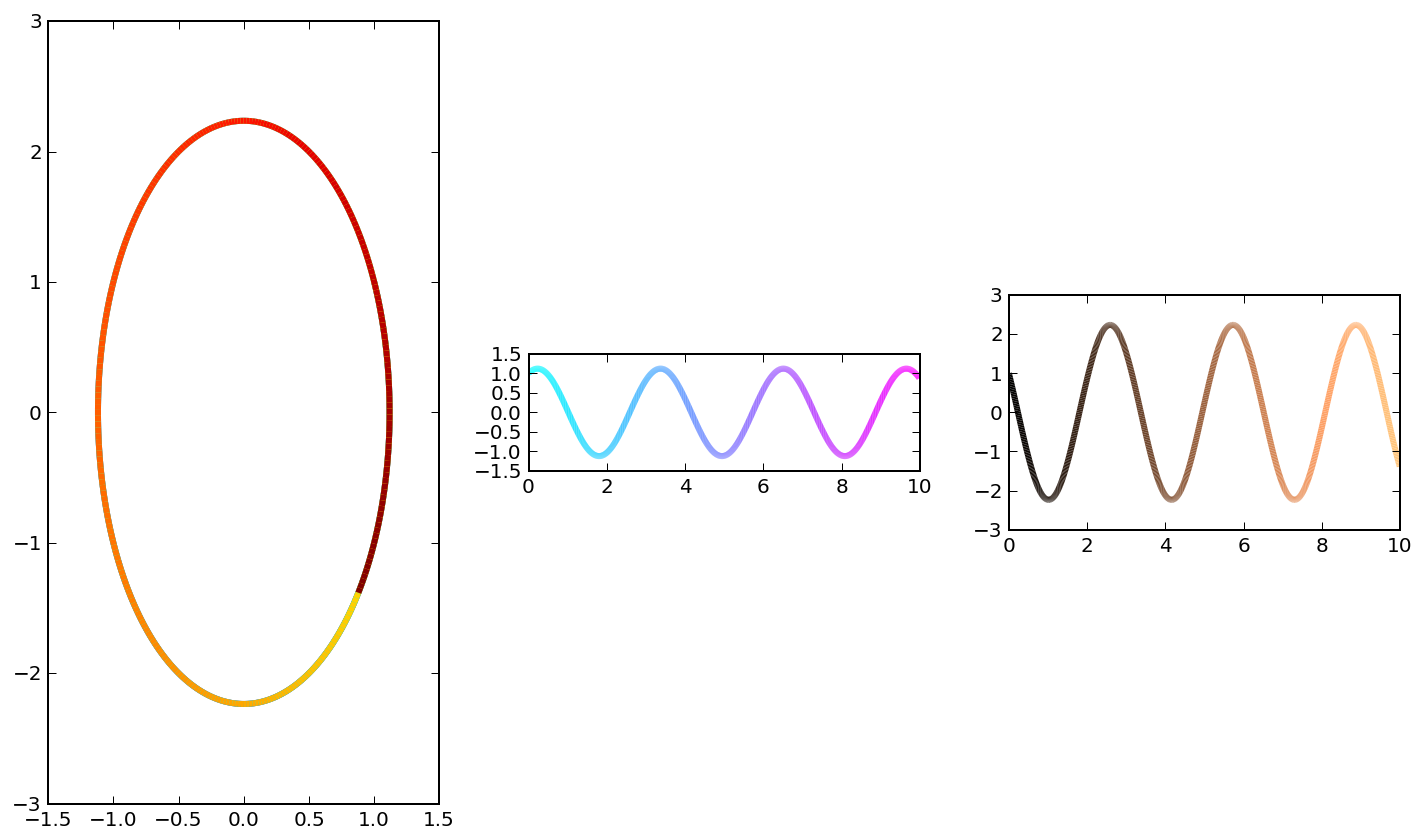In :
from numpy import zeros, linspace, array
from scipy.integrate import ode
from pylab import figure, show, xlabel, ylabel
from mpl_toolkits.mplot3d import Axes3D

def lorenz_sys(t, q):
x = q
y = q
z = q
# sigma, rho and beta are global.
f = [sigma * (y - x),
rho*x - y - x*z,
x*y - beta*z]
return f

ic = [1.0, 2.0, 1.0]
t0 = 0.0
t1 = 100.0
dt = 0.01

sigma = 10.0
rho = 28.0
beta = 10.0/3

solver = ode(lorenz_sys)

t = []
sol = []
solver.set_initial_value(ic, t0)
#solver.set_integrator('dop853')
solver.set_integrator('dopri5')

while solver.successful() and solver.t < t1:
solver.integrate(solver.t + dt)
t.append(solver.t)
sol.append(solver.y)

t = array(t)
sol = array(sol)

fig = figure()
ax = Axes3D(fig)
ax.plot(sol[:,0], sol[:,1], sol[:,2])
xlabel('x')
ylabel('y')
show()